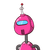# (3) if the first term of a G.P is 5 andcommon ratio is -5 then which termis 3125 ?o56O7<

(3) if the first term of a G.P is 5 and
common ratio is -5 then which term
is 3125 ?
o
5
6
O
7
8​

### 1 thought on “(3) if the first term of a G.P is 5 and<br />common ratio is -5 then which term<br />is 3125 ?<br />o<br />5<br />6<br />O<br />7<”

1.5 is correct answer for this question

Step-by-step explanation:

a=5 and r=-5

for gp

an=arn-¹

a.t.q

=5×-5^n-1=3125

-5^n-1=3125/5

-5^n-1=625

-5^n/5=625

-5^n=5⁴×5

-5^n=5⁵

so

bases are same so

exponent are same

so n=5 ans